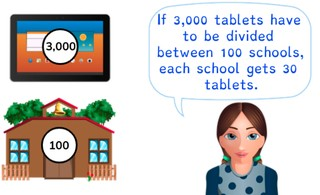Dividing a number greater than 1,000 by 10, 100, and 1,000

# Dividing a number greater than 1,000 by 10, 100, and 1,000

Students learn to divide a number greater than 1,000 by 10, 100, and 1,000.8,000 schools use Gynzy92,000 teachers use Gynzy1,600,000 students use Gynzy

## General

The students learn to divide a number greater than 1,000 by 10, 100, and 1,000.

## Standards

CCSS.Math.Content.4.NBT.A.1 / 4.NBT.B.6

## Learning objective

Students will be able to divide a number greater than 1,000 by 10, 100, or 1,000.

## Introduction

The students solve the division problems with numbers to 1,000 divided by 10.

## Instruction

Explain that when you divide by 10 you think about how many groups of 10 are in the given number. Show this in the problem 180 ÷ 10. Show that you determine how many groups of ten are in each number to calculate the problem. With 100 you think about how many groups of 100 are in the numbers, and with 1,000 you determine how many groups of 1,000 are in in the number. Have the students solve the following division problems on their own. Now show the problem 1,200 ÷ 10. Explain that nothing changes with your thinking, you simply decide if you are figuring out how many groups of 10, 100, or 1,000 are in the given number. After this, have the students solve the following problems. Show the story problem and walk the students through the steps. Next they solve two story problems on their own.

Check whether the students can divide a number greater than 1,000 by 10, 100, and 1,000 by asking the following question:
- What steps do you follow to solve the problem 52,000 ÷ 10?

## Quiz

The students test their understanding of dividing a number greater than 1,000 by 10, 100, and 1,000 through ten exercises. For some of the exercises the students are given possible answers to choose between, and for others they must provide the answer to a division problem on their own. Some of the exercises are story problems.

## Closing

Discuss once again the importance of being able to divide a number greater than 1,000 by 10, 100, and 1,000. As a closing activity have the students determine how many zeros belong at the end of each answer and drag the zeros to the right places.

## Teaching tips

Have students that have difficulty with dividing a number greater than 1,000 by 10, 100, or 1,000 first practice solving division problems with a number to 1,000 divided by 10.

### The online teaching platform for interactive whiteboards and displays in schools

• Save time building lessons

• Manage the classroom more efficiently

• Increase student engagement